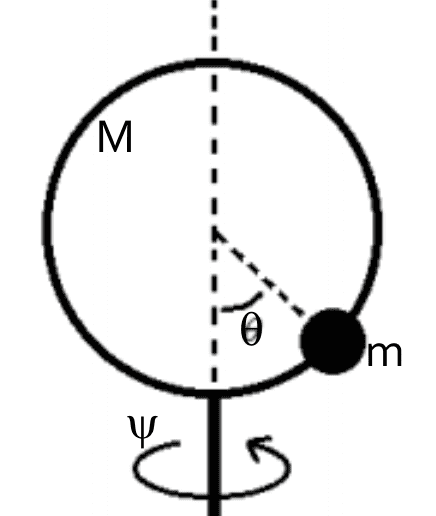# Something about configuration manifolds in classical mechanics

• A
• wrobel

#### wrobel

I think it could be interesting.

Consider a mechanical systemA circle of mass M can rotate about the vertical axis. The angle of rotation is coordinated by the angle ##\psi##. A bead of mass m>0 can slide along this circle. The position of the bead relative the circle is given by the angle ##\theta##.

It is interesting to note that if M>0 the Lagrangian of this system is defined on the tangent bundle ##T\mathbb{T}^2##. But if M=0 then the Lagrangian is defined on the tangent bundle ##TS^2##.

Last edited:
•martinbn

•